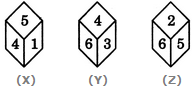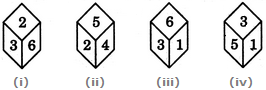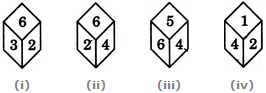# Non Verbal Reasoning - Cubes and Dice

### Exercise :: Cubes and Dice - Section 2

26.

Three different positions X, Y and Z of a dice are shown in the figures given below. Which number lies at the bottom face in position Y?A. 1 B. 2 C. 5 D. Cannot be determined

Answer: Option B

Explanation:

From positions X and Y we conclude that 1, 5, 6 and 3 lie adjacent to 4. Therefore, 2 must lie opposite 4. From positions Y and Z we conclude that 4, 3, 2 and 5 lie adjacent to 6. Therefore, 1 must lie opposite 6. Thus, 2 lies opposite 4, 1 lies opposite 6 and consequently 5 lies opposite 3.

As analysed above, the number on the face opposite 4 is 2. In position Y, since 4 lies on the top, therefore 2 must lie at the bottom face.

27.

What is the opposite 3, if four different positions of dice are as shown below?A. 6 B. 4 C. 3 D. 2

Answer: Option B

Explanation:

From figures (i), (iii) and (iv), we conclude that 2, 6, 1. and 5 appear adjacent to 3. Clearly, 4 will appear opposite 3.

28.

The four different positions of dice are given below: Which number is on the face opposite 6?A. 1 B. 2 C. 3 D. 4

Answer: Option A

Explanation:

From figures (i), (ii) and (iii), we conclude that the numbers 2, 3, 4 and 5 appear adjacent to 6.

Clearly, the remaining number i.e. 1 will appear on the face opposite 6.

29.

A dice is numbered from 1 to 6 in different ways.

If 1 is opposite to 2 and adjacent to 3 and 4, then which of the following statements is necessarily true?

 A. 2 is adjacent to 6 B. 3 is adjacent to 4 C. 4 is opposite to 6 D. 3 is opposite to 5

Answer: Option A

Explanation:

If 1 is opposite to 2, then 2 cannot lie opposite to any of the numbers 3, 4, 5 or 6. Hence, 2 necessarily lies adjacent to 6.

30.

Three different positions X, Y and Z of a dice are shown in the figures given below. Which number lies opposite 6?A. 1 B. 2 C. 4 D. 5

Answer: Option A

Explanation:

From positions X and Y we conclude that 1, 5, 6 and 3 lie adjacent to 4. Therefore, 2 must lie opposite 4. From positions Y and Z we conclude that 4, 3, 2 and 5 lie adjacent to 6. Therefore, 1 must lie opposite 6. Thus, 2 lies opposite 4, 1 lies opposite 6 and consequently 5 lies opposite 3.

As analysed above, 1 lies opposite 6.

#### Current Affairs 2021

Interview Questions and Answers